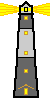# EPA On-line Tools for Site Assessment Calculation

The distribution of mass in a multiphase system depends on partitioning between the phases. The partition coefficients depend on properties of the chemical and the amounts of water, NAPL, air and organic carbon in the pore space.The mass distribution calculator only calculates the partitioning between between water, air, fuel and solids in the subsurface. Use the effective solubility calculator to determine the partitioning (concentrations) between only water and a fuel.

 Mass Distribution Calculator
 Mass Distribution Masswater = Cw Swq MassNAPL = Cw Ko Soq Massair = Cw Kh Saq Masssolid = Cw Kdrb Sw = Fraction of the pore space filled by water (water saturation) So = Fraction of the pore space filled by NAPL (NAPL saturation) Sa = Fraction of the pore space filled by air (air saturation) Ko = NAPL/water partition coefficient Kh = Henry's Law coefficient Kd = soil/water distribution coefficient Cw = Effective solubility q = porosity rb = bulk density Effective SolubilityCw = xo S Cw = Effective solubility xo = mole fraction (of chemical in fuel) S = solubility Mole Fraction xo = (estimate) ro / ( MWo S) ro = fuel density MWo = average molecular weight of fuel est. 105 g/mole for gasoline est. 165 g/mole for jet fuel est. 230 g/mole for diesel fuel

Site Name
Date
Distance Unit
Chemical
Solubility (S) mg/L
Optional Revised Solubility (S) mg/L
Example Fuel
Fuel composition note:
Average Molecular Weight of Fuel (MWo)
Percent Mass Fraction
Effective Solubility (Cw) mg/L
Porosity
Solids density
Fraction Organic Carbon Try 0.0001
Water Saturation Fraction of the pore space filled with water
NAPL Saturation Fraction of the pore space filled with NAPL
Phase %Mass Concentration
NAPL (Fuel) mg/L fuel
Water mg/L water
Air mg/L air
Solids mg/kg solids
Smear zone thickness